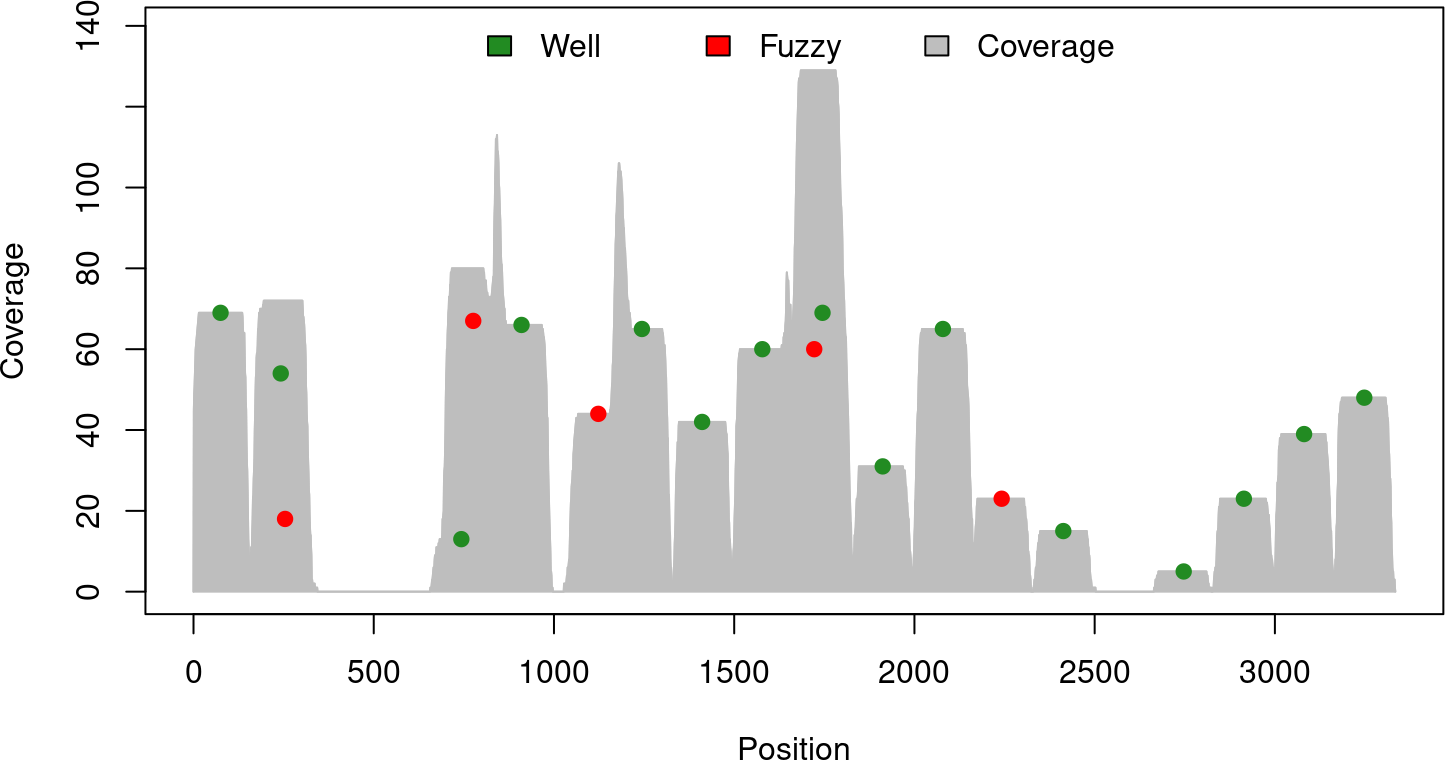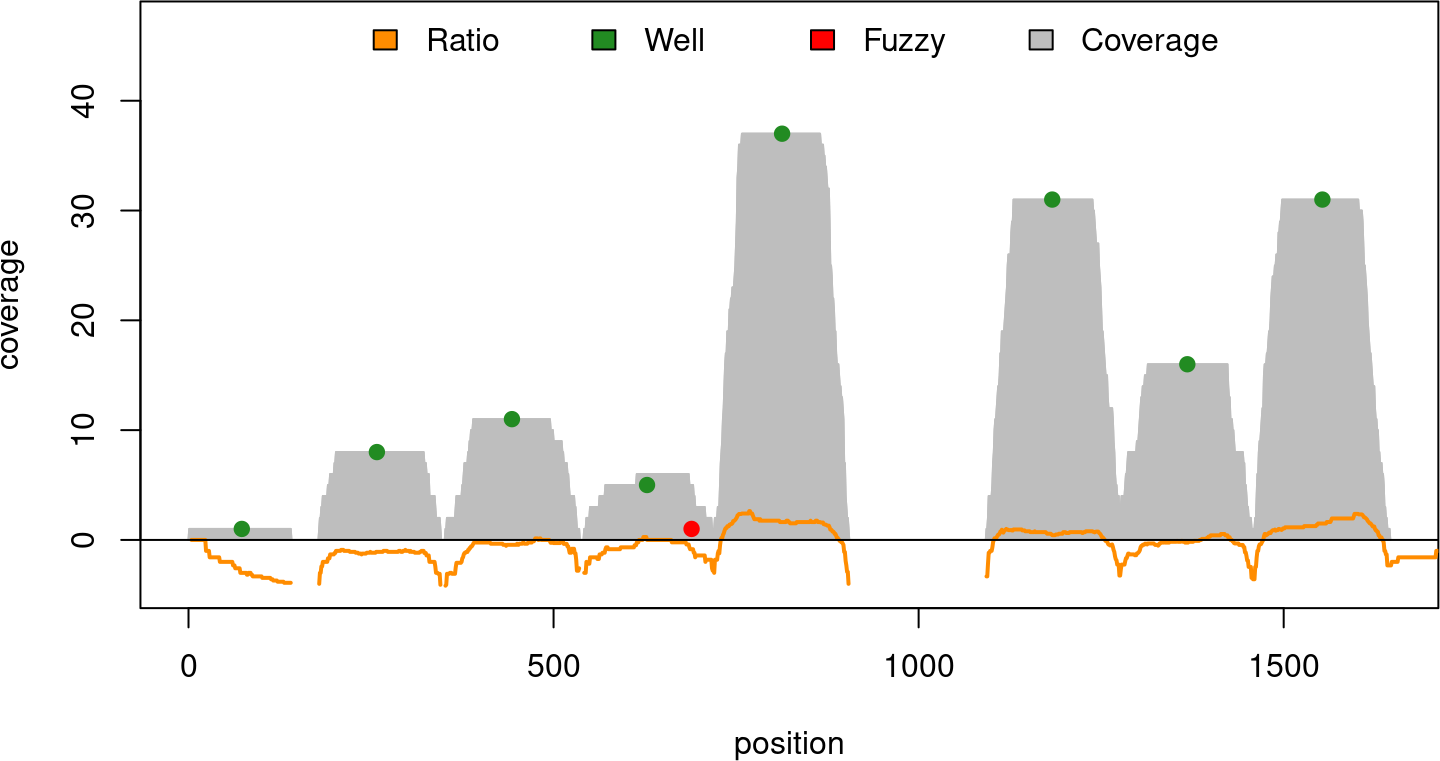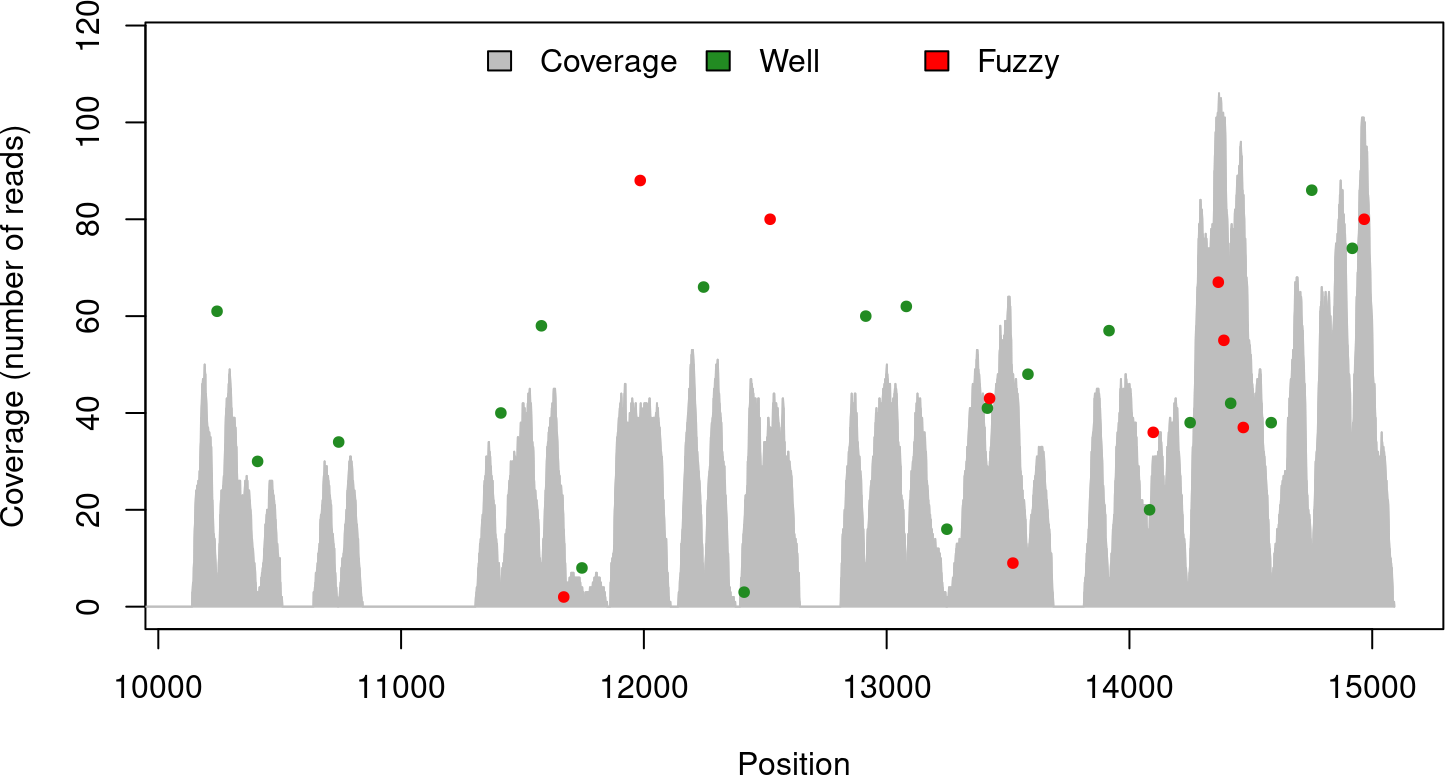# Contents

Package: nucleoSim
Authors: Rawane Samb [aut], Astrid Deschênes [cre, aut] (https://orcid.org/0000-0001-7846-6749), Pascal Belleau [aut] (https://orcid.org/0000-0002-0802-1071), Arnaud Droit [aut]
Version: 1.27.0
Compiled date: 2022-11-01

# 1 Licensing and citing

This package and the underlying nucleoSim code are distributed under the Artistic license 2.0. You are free to use and redistribute this software.

If you use this package for a publication, we would ask you to cite the following:

Samb R, Khadraoui K, Belleau P, et al. (2015). “Using informative Multinomial-Dirichlet prior in a t-mixture with reversible jump estimation of nucleosome positions for genome-wide profiling.” Statistical Applications in Genetics and Molecular Biology. Volume 14, Issue 6, Pages 517–532, ISSN (Online) 1544-6115, ISSN (Print) 2194-6302, December 2015. doi: 10.1515/sagmb-2014-0098

Flores O et Orozco M (2011). “nucleR: a package for non-parametric nucleosome positioning.” Bioinformatics, 27, pp. 2149–2150. doi: 10.1093/bioinformatics/btr345

# 2 Introduction

nucleoSim can simulate datasets for nucleosomes experiments.

The nucleoSim package generates synthetic maps with sequences covering nucleosome regions as well as synthetic maps with forward and reverse reads (paired-end reads) emulating next-generation sequencing. Furthermore, synthetic hybridization data of “Tiling Arrays” can also be generated.

The nucleoSim package allows the user to introduce various ‘contaminants’ into the sequence datasets, such as fuzzy nucleosomes and missing nucleosomes, in order to be more realistic and to enable the evaluation of the influence of common ‘noise’ on the detection of nucleosomes.

• Select the number of well-positioned nucleosomes to generate
• Select the number of well-positioned nucleosomes to randomly delete
• Select the number of fuzzy nucleosomes to add
• Select the type of distribution used to assign the start position to the sequences associated with the nucleosomes
• Select the variance associated with the distribution of the start position of the sequences for the well-positioned nucleosomes (or the start position of the forward reads for paired-end reads)
• Select the variance associated with the distribution of the start position of the sequences for the fuzzy nucleosomes (or the start position of the forward reads for paired-end reads)
• Select the variance associated with the Normal distribution used to assign the length of each sequence (or the distance between start positions of paired-end reads)
• Select the maximum sequence coverage associated with one nucleosome
• Select the length of the DNA linker
• Select the length of the nucleosomes (not recommended changing default value)
• Select a seed when results need to be reproducible

The nucleoSim package has been largely inspired by the Generating synthetic maps section of the Bioconductor nucleR package (Flores et Orozco, 2011).

As with any R package, the nucleoSim package should first be loaded with the following command:

library(nucleoSim)

# 4 Description

The packages can generate 2 types of synthetic data sets:

Synthetic Nucleosome Maps: A map with complete sequences covering the nucleosome regions

Synthetic Nucleosome Samples: A map with forward and reverse reads (paired-end reads) emulating those obtained using a next-generation sequencing technology on a nucleosome map

## 4.1 Synthetic Nucleosome Maps

A synthetic nucleosome map is a section of genome covered by a fixed number of nucleosomes. Each nucleosome being associated with a specific number of sequences. The parameters passed to the syntheticNucMapFromDist() function are going to affect the distribution of the nucleosomes, as well as, the sequences associated with each nucleosome.

Technically, the synthetic nucleosome map is separated into 3 steps:

The synthetic nucleosome map is split into a fixed number of sections (wp.num) of equal length ((nuc.len + lin.len) bases). The center of the nucleosomes is positioned at a fixed number of bases from the beginning of each section (round(nuc.len/2) bases). Sequences are assigned, to each nucleosome, using an uniform distribution. The number of sequences, assigned to each nucleosome, can vary from 1 to max.cover. The distribution (distr), as well as the variance (wp.var) are used to add some fluctuation to the starting position of the sequences, which as a mean position corresponding to the starting position of a region. Some fluctuation of the length of the sequence is also added following a normal distribution with a fixed variance (len.var). The mean length of the sequences corresponds to the length of the nucleosomes (nuc.len).

2. Deleting some well-positioned nucleosomes

A fixed number of nucleosomes (wp.del) are deleted. Each nucleosome has an equal probability to be deleted. A nucleosome is considered deleted when all sequences associated with it are eliminated.

A fixed number of fuzzy nucleosomes (fuz.num) are added. The position of the fuzzy nucleosomes is selected following an uniform distribution. Such as for the well-positioned nucleosomes, sequences are assigned, to each fuzzy nucleosome, using an uniform distribution. The number of sequences assigned can vary from 1 to max.cover. The distribution (distr), as well as the variance (wp.var) are used to add some fluctuation to the starting position of the sequences, which as a mean position corresponding to the starting position of a region. Some fluctuation of the length of the sequence is also added following a normal distribution with a fixed variance (len.var). The mean length of the sequences corresponds to the length of the nucleosomes (nuc.len).

### 4.1.1 Create a nucleosome map using syntheticNucMapFromDist()

This is an example showing how a synthetic nucleosome map can be generated.

wp.num           <- 20         ### Number of well-positioned nucleosomes
wp.del           <- 5          ### Number of well-positioned nucleosomes to delete
wp.var           <- 30         ### variance associated with the starting
###   position of the sequences of the
###   well-positioned nucleosomes
fuz.num          <- 5          ### Number of fuzzy nucleosomes
fuz.var          <- 50         ### Variance associated with the starting
###   positions of the sequences for the
###   fuzzy nucleosomes
max.cover        <- 70         ### Maximum sequences associated with one
###   nucleosome (default: 100)
nuc.len          <- 147        ### Length of the nucleosome (default: 147)
len.var          <- 12         ### variance associated with the length of
###   the sequences (default: 10)
lin.len          <- 20         ### Length of the DNA linker (default: 20)
distr            <- "Normal"   ### Type of distribution to use

rnd.seed         <- 210001     ### Set seed when result needs to be reproducible

#### Create a synthetic nucleosome map
nucleosomeMap <- syntheticNucMapFromDist(wp.num=wp.num, wp.del=wp.del,
wp.var=wp.var, fuz.num=fuz.num, fuz.var=fuz.var,
max.cover=max.cover, nuc.len=nuc.len, len.var=len.var,
lin.len=lin.len, rnd.seed=rnd.seed, distr=distr)

#### The start positions of all well-positioned nucleosomes
nucleosomeMap$wp.starts ##  1 168 669 836 1170 1337 1504 1671 1838 2005 2339 2673 2840 3007 3174 #### The number of sequences associated with each well-positioned nucleosome nucleosomeMap$wp.nreads
##   69 54 13 66 65 42 60 69 31 65 15  5 23 39 48

#### IRanges object containing all sequences for the well-positioned nucleosomes
head(nucleosomeMap$wp.reads, n = 2) ## IRanges object with 2 ranges and 0 metadata columns: ## start end width ## <integer> <integer> <integer> ##  3 144 142 ##  -1 154 156 #### The start positions of all fuzzy nucleosomes nucleosomeMap$fuz.starts
##  1049 2168  702  180 1648

#### The number of sequences associated with each fuzzy nucleosome
nucleosomeMap$fuz.nreads ##  44 23 67 18 60 #### A IRanges object containing all sequences for the fuzzy nucleosomes head(nucleosomeMap$fuz.reads, n = 2)
## IRanges object with 2 ranges and 0 metadata columns:
##           start       end     width
##       <integer> <integer> <integer>
##         1044      1190       147
##         1054      1198       145

#### A IRanges object containing all sequences for all nucleosomes
head(nucleosomeMap$syn.reads, n = 2) ## IRanges object with 2 ranges and 0 metadata columns: ## start end width ## <integer> <integer> <integer> ##  3 144 142 ##  -1 154 156 The synthetic nucleosome map can easily be visualized using plot() function. On the graph, each nucleosome is located on the graph using the coordonnates: (x,y) = (the central position of the nucleosome, the number of sequences associated with the nucleosome) #### Create visual representation of the synthetic nucleosome map plot(nucleosomeMap, xlab="Position", ylab="Coverage")### 4.1.2 Simulate hybridization data of Tiling Arrays The syntheticNucMapFromDist() function contains an option (as.ratio) which enable the simulation of hybridization data of “Tiling Arrays”. The data are generated by calculating the ratio between the nucleosome map and a control map of random sequences created using a uniform distribution. The control map simulates a DNA randomly fragmented sample. This is an example showing how a synthetic nucleosome map can be generated. as.ratio <- TRUE ### Activate the simulation of hybridization data rnd.seed <- 212309 ### Set seed when result needs to be reproducible #### Create a synthetic nucleosome map with hybridization data nucleosomeMapTiling <- syntheticNucMapFromDist(wp.num=10, wp.del=2, wp.var=20, fuz.num=1, fuz.var=32, max.cover=50, nuc.len=145, len.var=3, lin.len=40, rnd.seed=rnd.seed, as.ratio=as.ratio, distr="Uniform") #### Control sequences for hybridization data (only when as.ratio = TRUE) head(nucleosomeMapTiling$ctr.reads, n=4)
## IRanges object with 4 ranges and 0 metadata columns:
##           start       end     width
##       <integer> <integer> <integer>
##          273       511       239
##          361       610       250
##         1266      1473       208
##          460       622       163

#### Ratio for hybridization data (only when as.ratio = TRUE)
head(nucleosomeMapTiling$syn.ratio, n=4) ## numeric-Rle of length 4 with 2 runs ## Lengths: 3 1 ## Values : NA 0 #### Create visual representation of the synthetic nucleosome map plot(nucleosomeMapTiling)## 4.2 Synthetic Nucleosome Samples A synthetic nucleosome sample is a map with forward and reverse reads (paired-end reads) emulating those obtained using a next-generation sequencing technology. It is created using the same first 3 steps than the synthetic nucleosome map. However, some new steps are present: 1. Adding well-positioned nucleosomes The synthetic nucleosome map is split into a fixed number of sections (wp.num) of equal length ((nuc.len + lin.len) bases). The center of the nucleosomes are positioned at a fixed number of bases from the beginning of each section (round(nuc.len/2) bases). Paired-end reads are assigned, to each nucleosome, using an uniform distribution. The number of paired-end reads, assigned to each nucleosome, can vary from 1 to max.cover. The distribution (distr), as well as the variance (wp.var) are used to add some fluctuation to the starting position of the forward reads, which as a mean position corresponding to the starting position of a region. Some fluctuation of the distance between start positions of paired-end reads is added following a normal distribution with a fixed variance (len.var). The mean distance between start positions of paired-end reads corresponds to the length of the nucleosomes (nuc.len). 2. Deleting some well-positioned nucleosomes A fixed number of nucleosomes (wp.del) are deleted. Each nucleosome has an equal probability to be deleted. A nucleosome is considered deleted when all paired-end reads associated with it are eliminated. 3. Adding fuzzy nucleosomes A fixed number of fuzzy nucleosomes (fuz.num) are added. The position of the fuzzy nucleosomes is selected following an uniform distribution. Such as for the well-positioned nucleosomes, reads are assigned, to each fuzzy nucleosome, using an uniform distribution. The number of paired-end reads assigned can vary from 1 to max.cover. The distribution (distr), as well as the variance (wp.var) are used to add some fluctuation to the starting position of the forward reads, which as a mean position corresponding to the starting position of a region. Some fluctuation of the distance between start positions of paired-end reads is also added following a normal distribution with a fixed variance (len.var). The mean distance between start positions of paired-end reads corresponds to the length of the nucleosomes (nuc.len). All reads have a fixed length (read.len). 4. Adding an offset An offset (offset) is added to all nucleosomes and reads positions to ensure that all values are positive (mainly pertinent for reads). ### 4.2.1 Create a nucleosome sample using syntheticNucReadsFromDist() This function needs information about the nucleosomes and their distribution to generate a nucleosome sample. The output is of class syntheticNucMap. wp.num <- 30 ### Number of well-positioned nucleosomes wp.del <- 10 ### Number of well-positioned nucleosomes ### to delete wp.var <- 30 ### variance associated with the starting ### positions of the sequences for the ### well-positioned nucleosomes fuz.num <- 10 ### Number of fuzzy nucleosomes fuz.var <- 50 ### Variance associated with the starting ### positions of the sequences for the ### fuzzy nucleosomes max.cover <- 90 ### Maximum paired-end reads associated with ### one nucleosome (default: 100) nuc.len <- 147 ### Length of the nucleosome (default: 147) len.var <- 12 ### variance associated with the distance ### between start positions of ### paired-end reads (default: 10) lin.len <- 20 ### Length of the DNA linker (default: 20) read.len <- 45 ### Length of the generated forward and ### reverse reads (default: 40) distr <- "Uniform" ### Type of distribution to use offset <- 10000 ### The number of bases used to offset ### all nucleosomes and reads rnd.seed <- 202 ### Set seed when result needs to be ### reproducible #### Create Uniform sample nucleosomeSample <- syntheticNucReadsFromDist(wp.num=wp.num, wp.del=wp.del, wp.var=wp.var, fuz.num=fuz.num, fuz.var=fuz.var, max.cover=max.cover, nuc.len=nuc.len, len.var=len.var, read.len=read.len, lin.len=lin.len, rnd.seed=rnd.seed, distr=distr, offset=offset) #### The central position of all well-positioned nucleosomes with the #### number of paired-end reads each associated with each one head(nucleosomeSample$wp, n = 2)
## 1     10242     61
## 2     10409     30

#### The central position of all fuzzy nucleosomes with the
#### number of paired-end reads each associated with each one
head(nucleosomeSample$fuz, n = 2) ## nucleopos nreads ## 1 11985 88 ## 2 14098 36 #### A data.frame with the name of the synthetic chromosome, the starting #### position, the ending position and the direction of all forward and #### reverse reads head(nucleosomeSample$dataIP, n = 2)
##             chr start   end strand ID
## 1 chr_SYNTHETIC 10140 10185      + 11
## 2 chr_SYNTHETIC 10140 10185      + 15

The synthetic nucleosome sample can easily be visualized using plot() function. On the graph, each nucleosome is located on the graph using the coordinates:

(x,y) = (the central position of the nucleosome, the number of paired-end reads associated with the nucleosome)

#### Create visual representation of the synthetic nucleosome sample
plot(nucleosomeSample, xlab="Position", ylab="Coverage (number of reads)")### 4.2.2 Create a nucleosome sample using syntheticNucReadsFromMap()

A synthetic nucleosome sample can be created using a nucleosome map. The nucleosomes and reads present in the nucleosome map will be added an offset. Forward and reverse reads will also be generated. The output is of class syntheticNucMap.


#### A nucleosome map has already been created
class(nucleosomeMap)
##  "syntheticNucMap"

####
read.len    <- 45   ### The length of the reverse and forward reads
offset      <- 500  ### The number of bases used to offset all nucleosomes and reads

#### Create nucleosome sample

#### A data.frame with the name of the synthetic chromosome, the starting
#### position, the ending position and the direction of all forward and
##             chr start end strand ID
## 1 chr_SYNTHETIC   490 535      + 10
## 2 chr_SYNTHETIC   490 535      + 23

# 5 Session info

Here is the output of sessionInfo() on the system on which this document was compiled:

## R Under development (unstable) (2022-10-25 r83175)
## Platform: x86_64-pc-linux-gnu (64-bit)
## Running under: Ubuntu 22.04.1 LTS
##
## Matrix products: default
## BLAS:   /home/biocbuild/bbs-3.17-bioc/R/lib/libRblas.so
## LAPACK: /usr/lib/x86_64-linux-gnu/lapack/liblapack.so.3.10.0
##
## locale:
##   LC_CTYPE=en_US.UTF-8       LC_NUMERIC=C
##   LC_TIME=en_GB              LC_COLLATE=C
##   LC_MONETARY=en_US.UTF-8    LC_MESSAGES=en_US.UTF-8
##   LC_PAPER=en_US.UTF-8       LC_NAME=C
##  LC_MEASUREMENT=en_US.UTF-8 LC_IDENTIFICATION=C
##
## attached base packages:
##  stats     graphics  grDevices utils     datasets  methods   base
##
## other attached packages:
##  nucleoSim_1.27.0 knitr_1.40       BiocStyle_2.27.0
##
## loaded via a namespace (and not attached):
##   cli_3.4.1           magick_2.7.3        rlang_1.0.6
##   xfun_0.34           stringi_1.7.8       highr_0.9
##   jsonlite_1.8.3      S4Vectors_0.37.0    htmltools_0.5.3
##  sass_0.4.2          stats4_4.3.0        rmarkdown_2.17
##  evaluate_0.17       jquerylib_0.1.4     fastmap_1.1.0
##  yaml_2.3.6          IRanges_2.33.0      bookdown_0.29
##  BiocManager_1.30.19 stringr_1.4.1       compiler_4.3.0
##  Rcpp_1.0.9          digest_0.6.30       R6_2.5.1
##  magrittr_2.0.3      bslib_0.4.0         tools_4.3.0
##  BiocGenerics_0.45.0 cachem_1.0.6